Next: Semantic Security Up: Probabilistic Encryption Previous: Knowing a pseudo-square does   Contents

## The inability to distinguish two plaintexts

Theorem 5.1 of the paper addresses the issue we mentioned at the beginning of section 7. It shows that if we have an algorithm which can identify messages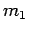and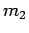and efficiently tell the difference between an encryption ofand an encryption of, then we could construct an algorithm which efficiently distinguishes squares from pseudo-squares. Thus (QRA) implies we cannot tell the difference betweenand.

Proof:11 Suppose we are trying to decide whether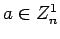is a square and that the two distinguishable messages areChoose 8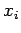randomly and consider the sequences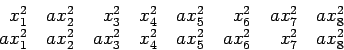If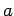is a pseudo-square, these will be randomly chosen encodings ofand. In this case, the performance of our assumed algorithm on the two sequences (averaged over repeated random choices of) will be different. Ifis a square, both sequences will be randomly chosen encodings of the message consisting of all 0's, so the algorithm's response on average to the two sequences will be identical.

#### Footnotes

...Proof:11
The argument we give is a simplification of the one in the paper, in that we do not use the sampling walk.'' The more complicated argument seems to be necessary to analyze encryption systems in general, as opposed to those based on squares and pseudo-squares.Next: Semantic Security Up: Probabilistic Encryption Previous: Knowing a pseudo-square does   Contents
Translated from LaTeX by Scott Sutherland
2002-12-14